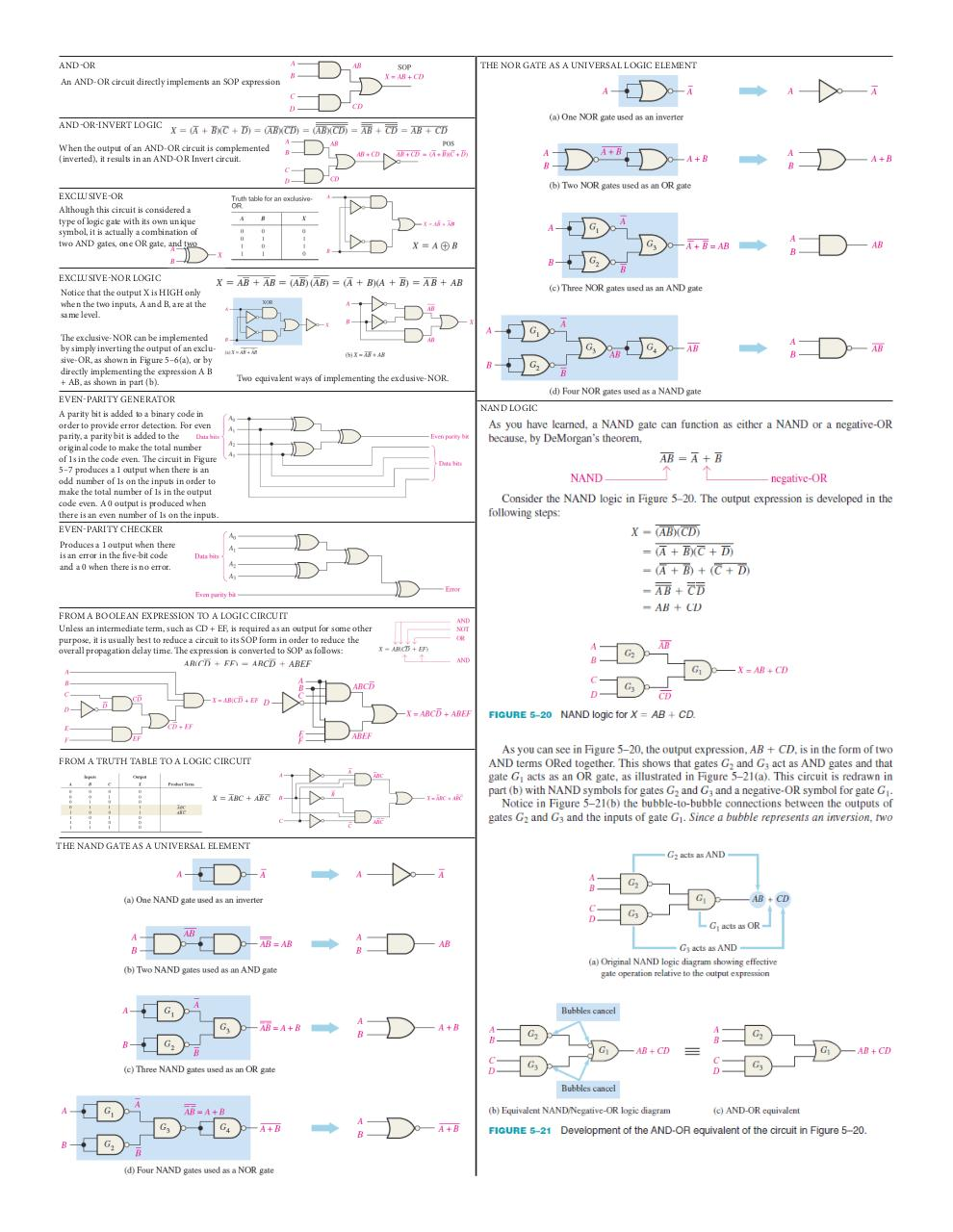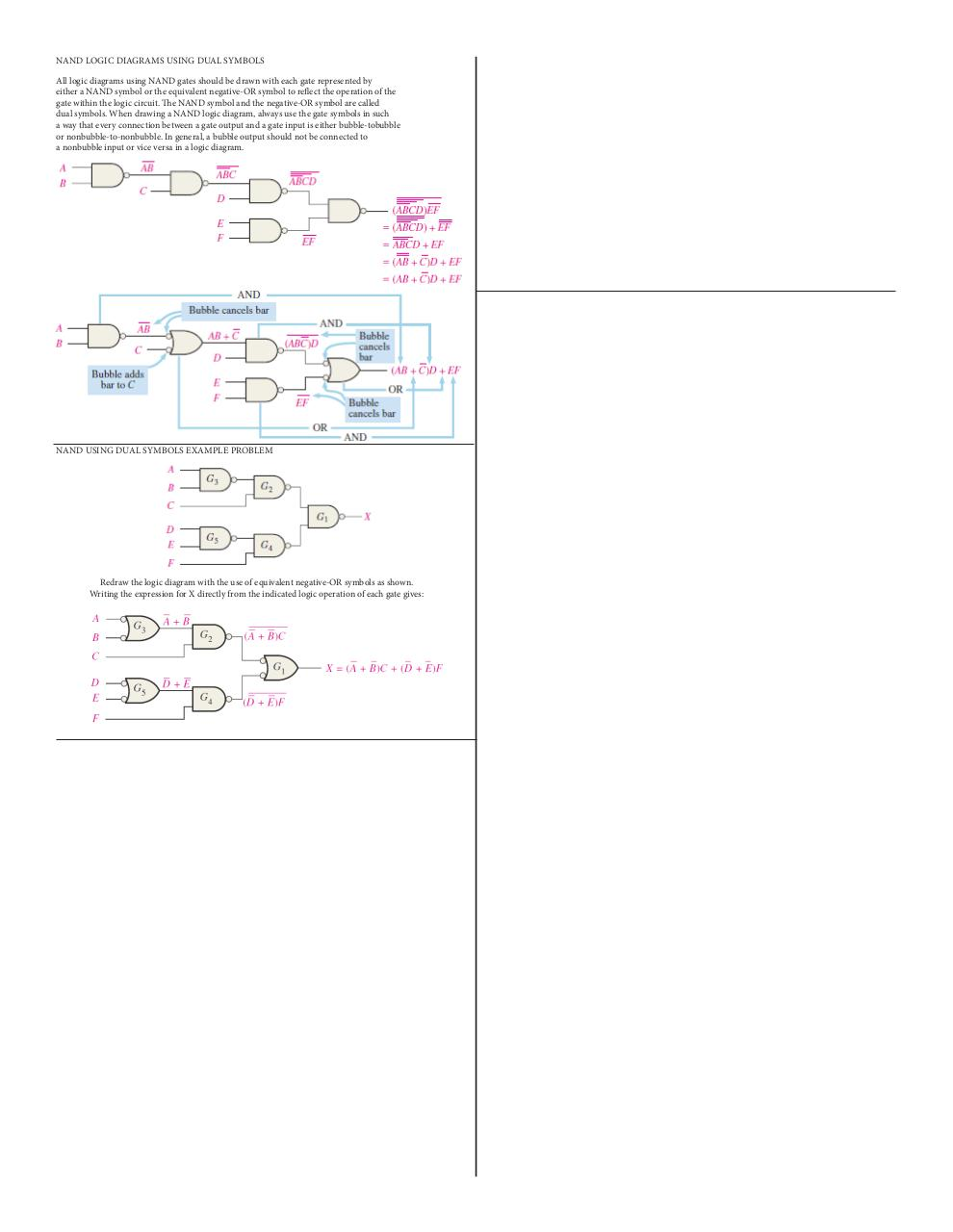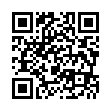# Cheat Sheet 3 .pdf

### File information

Original filename: Cheat Sheet 3.pdf

This PDF 1.4 document has been generated by Adobe InDesign CS6 (Windows) / Adobe PDF Library 10.0.1, and has been sent on pdf-archive.com on 20/10/2015 at 05:35, from IP address 73.1.x.x. The current document download page has been viewed 946 times.
File size: 336 KB (2 pages).
Privacy: public file

Cheat Sheet 3.pdf (PDF, 336 KB)

### Document preview

AND-OR

THE NOR GATE AS A UNIVERSAL LOGIC ELEMENT

An AND-OR circuit directly implements an SOP expression

AND-OR-INVERT LOGIC
When the output of an AND-OR circuit is complemented
(inverted), it results in an AND-OR Invert circuit.

EXCLUSIVE-OR
Although this circuit is considered a
type of logic gate with its own unique
symbol, it is actually a combination of
two AND gates, one OR gate, and two
EXCLUSIVE-NOR LOGIC
Notice that the output X is HIGH only
when the two inputs, A and B, are at the
same level.
The exclusive-NOR can be implemented
by simply inverting the output of an exclusive-OR, as shown in Figure 5–6(a), or by
directly implementing the expression A B
+ AB, as shown in part (b).

Two equivalent ways of implementing the exclusive-NOR.

EVEN-PARITY GENERATOR
A parity bit is added to a binary code in
order to provide error detection. For even
parity, a parity bit is added to the
original code to make the total number
of 1s in the code even. The circuit in Figure
5–7 produces a 1 output when there is an
odd number of 1s on the inputs in order to
make the total number of 1s in the output
code even. A 0 output is produced when
there is an even number of 1s on the inputs.
EVEN-PARITY CHECKER
Produces a 1 output when there
is an error in the five-bit code
and a 0 when there is no error.

FROM A BOOLEAN EXPRESSION TO A LOGIC CIRCUIT
Unless an intermediate term, such as CD + EF, is required as an output for some other
purpose, it is usually best to reduce a circuit to its SOP form in order to reduce the
overall propagation delay time. The expression is converted to SOP as follows:

FROM A TRUTH TABLE TO A LOGIC CIRCUIT

THE NAND GATE AS A UNIVERSAL ELEMENT

NAND LOGIC

NAND LOGIC DIAGRAMS USING DUAL SYMBOLS
All logic diagrams using NAND gates should be drawn with each gate represented by
either a NAND symbol or the equivalent negative-OR symbol to reflect the operation of the
gate within the logic circuit. The NAND symbol and the negative-OR symbol are called
dual symbols. When drawing a NAND logic diagram, always use the gate symbols in such
a way that every connection between a gate output and a gate input is either bubble-tobubble
or nonbubble-to-nonbubble. In general, a bubble output should not be connected to
a nonbubble input or vice versa in a logic diagram.

NAND USING DUAL SYMBOLS EXAMPLE PROBLEM

Redraw the logic diagram with the use of equivalent negative-OR symbols as shown.
Writing the expression for X directly from the indicated logic operation of each gate gives: